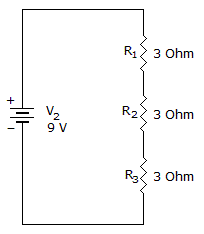# Electronics - Series Circuits - Discussion

### Discussion :: Series Circuits - General Questions (Q.No.3)

3.

If the resistance total in a series circuit doubles, current will:

 [A]. be the same [B]. be doubled [C]. reduce source voltage [D]. be halved

Explanation:

No answer description available for this question.

 Rakesh Mohapatra said: (Apr 24, 2011) As v=ir,so v=1/2(i)*(2r) so current is halved

 Anuja Shelke said: (May 24, 2011) i=v/r & if the resistance is doubled then the current will be i=v/2r. which is half of the previous.

 Arun Jain said: (Aug 25, 2011) By Ohm's Law Voltage(V) is directly praprotional to Current(C), and by Ohm's R is a praprotionality Constant, So In a series circuit voltage is constant(we can say V = 1),than By eq. V = IR 1 = IR (V=1) I = 1/R If R = 2 Then I = ? I = 1/2 that is Halfed

 Kotesh said: (Dec 25, 2011) In series total resistance value is 3+3+3=9 ohm's. supose in that circuit voltage is 9v. given data R=9 ohm's V=9v, simlarly R=2R (given conditions), so 2*9=18 ohms V=IR I=V/R I=9/18 1/2 that is Halfed

 Ahammed Najeeb said: (Jan 6, 2012) For example we can take a circuit given below.In this series total resistance value is 3+3+3=9 ohm's. supose in that circuit voltage is 9v. Given data R=9 ohm's V=9v, Simlarly R=2R (given conditions), so 2*9=18 ohms V=IR I=V/R I=9/18 1/2 That is halved.

 Sreeyush Sudhakaran said: (Feb 18, 2012) Case 1: V=IxR case 2: V=I'x2R Since Voltage source in both circuit is same IxR=I'x2R so I'=I/2 Current is halfed in second case

 Nagaraj Reddy said: (Oct 25, 2013) We know Ohm's law V=IR. If R is doubled then. V = I(2R). then, I= V/2R. I=(1/2)(V/R). Hence Current Halved.

 Rooshan Ashfaq said: (Jan 28, 2014) V=IR. I=V/R or R=V/I. As we see current is inversely proportional to resistance so value of current remain half if value of resistance I double.

 Bhabani Shankar Behera said: (Dec 3, 2015) R = 2. V = 1. I = V/R. So I = 1/2.

 Hari said: (Mar 31, 2018) Explain it in detail.

 Janvence said: (Jun 9, 2018) Thank you all for explaining it.